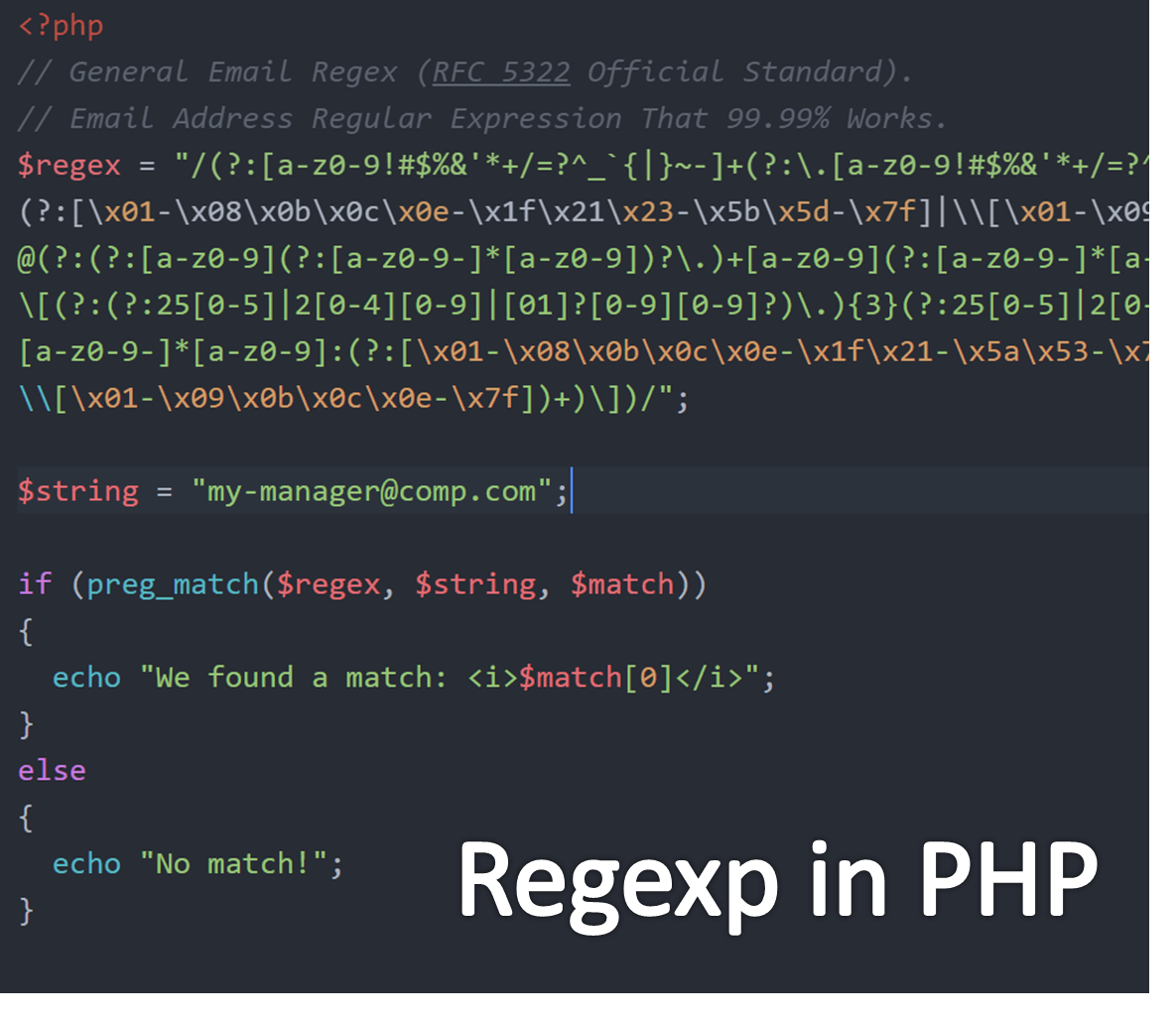← Back to all posts
Multiple operators

I could use some help with this. I have it set up so you can only input 2 numbers. I would like to have it so you could input something like 5+5 instead of having to say first number=5 and second number=5, then make the operation add. I tried putting this into a variable and printing it, but it only printed 5+5, instead of the result=10. Can I get some help with this? Thanks.

theangryepicbanana (1628)

I haven't used PHP before, but I'm sure you could do something using regex

Baconman321 (81)

@theangryepicbanana Could you explain to me what regex is and how to use it?

Baconman321 (81)

@Giothecoder yes but how would I use that in php? Like what would I write down?

Giothecoder (130)

Regular expressions in PHP
preg_match – this function is used to perform a pattern match on a string. It returns true if a match is found and false if a match is not found. preg_replace – this function is used to perform a pattern match on a string and then replace the match with the specified text.That should help idk.

Baconman321 (81)

@Giothecoder Can you break it down, since I do not understand this at all?

Baconman321 (81)

@theangryepicbanana Thanks so much, I get it now. However, how does that help me with operators. I need to have the variables understand operators in a string, not find or replace them.

theangryepicbanana (1628)

@Baconman321 well if you use capture groups (`a(.)c`), then you can just use the values from the matched groups. I'm not sure how it works in PHP, but it shouldn't be too difficult

Baconman321 (81)

@theangryepicbanana So exactly how would I do this? Like in an expression. Replace +,/,*, or - I find and add it to the variable?

theangryepicbanana (1628)

@Baconman321 basically if you were to match the input `1 + 2` with the regex `\s*([\+\-]?\d+)\s*([\+\-\*\/])\s*([\+\-]\d+)\s*`, you would get `1` as the first group, `+` as the second group, and then `3` as the third group.

malvoliothegood (727)

You could use the PHP `explode` function. I have not tried out this code, but it should work:

``````// \$data's value is sent through via a form...
\$data = "12 + 10";
// \$first is "12", \$second is "10", \$op is "+"
// Split string up using the space character
list(\$first, \$op, \$second) = explode(" ", \$data);
// Convert number strings to floats (decimals) and reassign to
// variables
\$first = floatval(\$first);
\$second = floatval(\$second);
\$result = NULL;
switch(\$op) {
case "+": \$result = \$first + \$second;
break;
case "-": \$result = \$first - \$second;
break;
case "*": \$result = \$first * \$second;
break;
case "/": \$result = \$first / \$second;
break;
default: echo "Incorrect operator. Try again...";
}
if (\$result != NULL) {
echo "The result is: \$result";
}``````